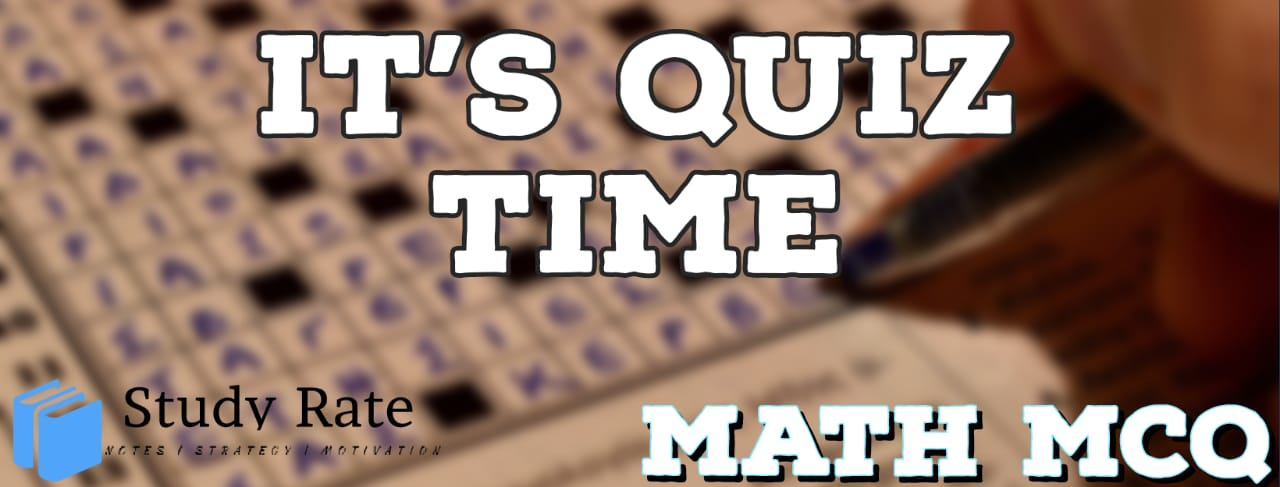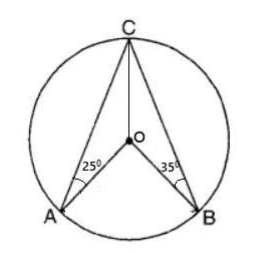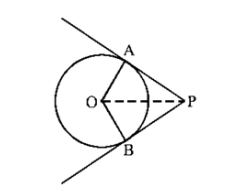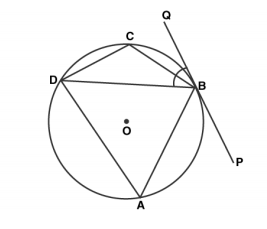## CBSE Class 10 Maths Chapter 10 – Circles MCQ Quiz

Free PDF Download of CBSE Class 10 Maths Chapter 10 – Circles Multiple Choice Questions with Answers. MCQ Questions for Class 10 Maths with Answers was Prepared Based on Latest Exam Pattern. Students can solve Class 10 Maths Circles MCQs with Answers to know their preparation level

CBSE Class 10 Maths Chapter 10- Circle

1. If angle between two radii of a circle is 130º, then the angle between the tangents at the ends of the radii is

2. The length of tangent from an external point P on a circle with centre O is

3. Three circles touch each other externally. The distance between their centres is 5 cm, 6cm and 7 cm. Find the radii of the circles.

4. A point P is 13 cm from the centre of the circle. The length of the tangent drawn from P to the circle is 12cm. Find the radius of the circle.

5. In the adjoining figure ‘O’ is the center of circle, ∠CAO = 25° and ∠CBO = 35°. What is the value of ∠AOB?6. In the following figure, if O is the centre of a circle, PQ is a chord and the tangent PR at P makes an angle of 50° with PQ, then ∠POQ is equal to7. In the following figure, PA and PB are tangents from a point P to a circle with centre O. Then the quadrilateral OAPB must be a8. ABCD is a cyclic quadrilateral PQ is a tangent at B. If ∠DBQ = 65°, then ∠BCD is9. In a circle of radius 5 cm, AB and AC are the two chords such that AB = AC = 6 cm. Find the length of the chord BC

10. A tangent PQ at a point P of a circle of radius 5 cm meets a line through the center O at a point Q such that OQ =13 cm. Length PQ is: